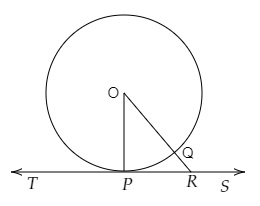# Prove that the perpendicular at the point of contact to the tangent to a circle passes through the centre of the circle.

To do:

We have to prove that the perpendicular at the point of contact to the tangent to a circle passes through the centre of the circle.

Solution:Let $TS$ be a tangent to the circle with centre $O$ at $P$.

Join $OP$.

Draw a line $OR$ which intersects the circle at $Q$ and meets the tangent $TS$ at $R$.

$OP = OQ$   (radii of the circle)

$OQ$\Rightarrow OP

Similarly,

$OP$ is less than all those lines which can be drawn from $O$ to $TS$.

$OP$ is the shortest such line.

Therefore,

$OP$ is perpendicular to $TS$.

Perpendicular through $P$ will pass through the centre of the circle.

Hence proved.

Updated on: 10-Oct-2022

38 Views